# Imaginary and Complex Numbers

Let’s start with the following calculation: $-2 = (-8)^{1/3} = (-8)^{2/6} = ((-8)^2)^{1/6} = 64^{1/6} = 2$.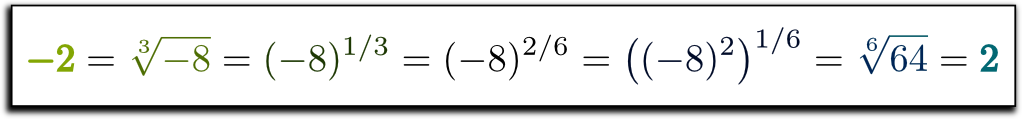This must be wrong… but I don’t see why!

The brutal answer would be that you should never write $a^p$ when $a$ is negative… But, this would conceal some wonderful underlying mathematics, including and especially the weirdness and awesomeness of imaginary and complex numbers! Let’s discover these mathematical objects in this article! Our approach will be highly geometric and, I think, much more insightful than the one you have learned (or will learn) at school.

## The Geometry of $n$-th Root

To understand what just happened, let’s focus on the first equality, namely $-2 = \sqrt{-8}$. It reads “$-2$ is the cube root of $-8$”. But what does that mean?

Doesn’t it mean that $(-2)^3 = -8$?

Yes! But, let’s have a geometrical understanding of what you’ve just said! To do so, notice that if we multiply both sides of the equality by any number, then the equality still holds. Indeed, if we multiply by $1$ both sides we obtain $1 \times (-2)^3 = 1 \times (-8)$, and if we multiply by $241$, we have $241 \times (-2)^3 = 241 \times (-8)$. More generally, if $x$ represents any number, we have $x \times (-2)^3 = x \times (-2) \times (-2) \times (-2) = x \times (-8)$. Thus, we can now see the operation “$\times (-2)$” as an operation on numbers, which, when applied three times, is equivalent to the operation “$\times (-8)$”!

I’m still not sure where you’re going with it…

Here’s the awesome part. These operations correspond to geometrical transformations made to the number line (they’re symmetries)! For instance, multiplying by $(-2)$ corresponds to inverting it, and stretching it by a factor 2. This is what’s done below three times!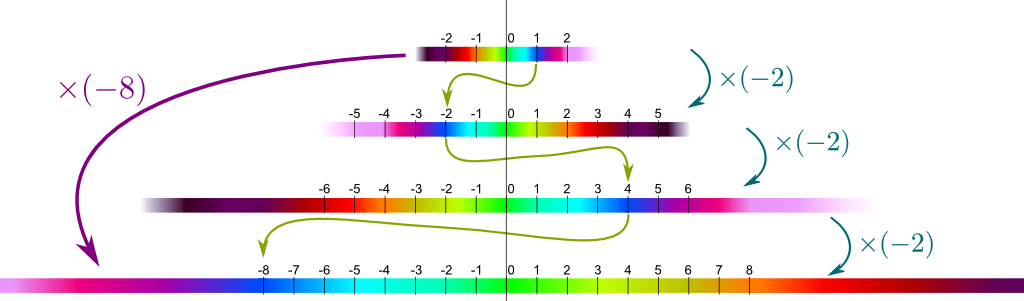It might look nice, but I don’t see the point of the geometrical approach…

Be patient! Let’s simplify the problem a little bit and consider the equality $-1 = (-1)^{1/3}$. What does it mean geometrically?

Well, I guess that “$\times (-1)$” corresponds to just inverting the number line, doesn’t it?

Yes! Now, this means that we can translate the algebraic relation $-1=(-1)^{1/3}$ by the geometrical phrase: Inverting the number line three times is equivalent to inverting it once. Sweet, isn’t it?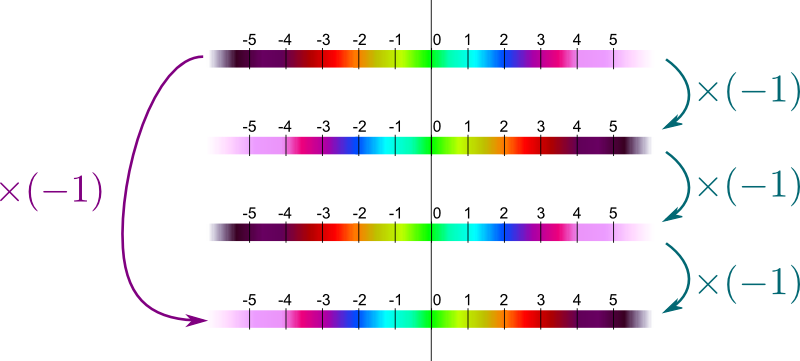Yes! But I still don’t see the point…

Hehe! The key idea of complex numbers lies in the next question… Is $(-1)$ the only one cube root of $(-1)$?

I think so… If you take a positive number, its cube will be positive… So the only number that works is $-1$…

Don’t think numerically! The whole point of my construction was to consider the problem geometrically!

Humm…

In other words, is there a geometrical operation on the number line, which, when applied three times, corresponds to inverting it?

Humm…

Come on! You can find it!

I know! How about rotating the number line by a 6th of a turn?

Bingo!

In fact, there are two such 6th-of-a-turn operations, depending on whether the turn is clockwise or anti-clockwise. Below are described these two operations, each applied three times to the number line.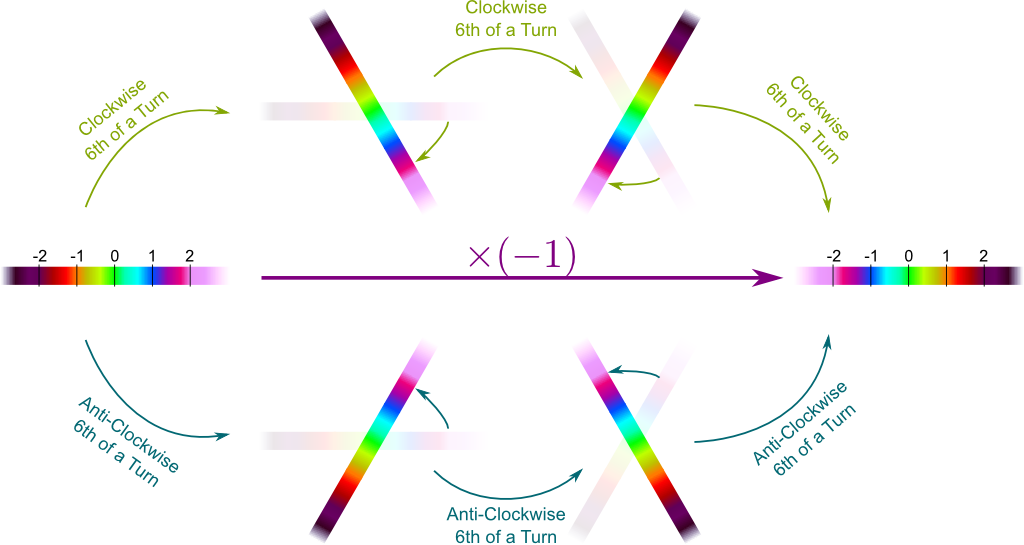In addition to the “$\times (-1)$” operation, this gives us a total of three cube roots of $(-1)$!

Does $(-8)$ have several cube roots too? What about the 6th roots of $64$?

Great questions! In fact, you should try to answer by yourself!

Humm… I guess a cube root of $(-8)$ can be obtained by a 6th of a turn (like $(-1)$), combined with a stretching of the number line, can’t it?

Exactly! Similarly, 6th roots of $64$ include dilations by a factor 2 combined with a rotation of one or two 6th of turn, clockwise or anti-clockwise. Plus, there are also operations “$\times (-2)$” and “$\times 2$”. This gives us six 6th roots of $64$. And as you can guess (or prove!), more generally, any number has $n$ $n$-th roots!

I’ve heard about the square root of $(-1)$… Is it obtained similarly to what we’ve done??

Once again, you should be the one who gives me the answer!

Humm…

In other words, is there a geometrical transformation which, when applied twice to the number line, is equivalent to simply inverting it?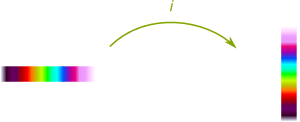I know! Rotations of a quarter of a turn!

There you go! By convention, we refer to the anti-clockwise quarter-of-a-turn rotation as $i$. This $i$ is so important that we have given it different names… which I all dislike! It’s known as the imaginary number (imaginary? number?), the square root of $(-1)$, or, worst of all, $\sqrt{-1}$.

What’s wrong with $\sqrt{-1}$?

What’s very wrong is that $i$ is not the only square root of $(-1)$. The clockwise quarter-of-a-turn rotation is a square root of $(-1)$ too! Plus, if you can’t write $\sqrt{-8}$, then you definitely can’t write $\sqrt{-1}$!

OK… That’s cool but I don’t see how this fixes the paradox of the introduction!

Hehe… We can now answer that elegantly!

The major flaw lies in the non-unicity of the $n$-th roots. This is what 19th-century French mathematician Évariste Galois called the ambiguity of $n$-th roots. More precisely, there’s not an actual unicity of the cube root of $(-8)$, nor is there a unicity of the 6th root of $64$. In particular, $(-2)$ is a 6th root of $64$, but it’s just not the one we refer to by $\sqrt{64}$.

Still, there’s a strong relation between cube roots of $(-8)$ and 6th roots of $64$, isn’t there?

Yes. And the other flaw of the formula of the introduction lies in the relation $(-8)^{1/3} = ((-8)^2)^{1/6}$. Literally, it says that a cube root of $(-8)$ is a sixth root of the square of $(-8)$.

Humm… I’m not sure I understand…

Once again, our salvation will come from geometry! Geometrically, this says that an operation which is equivalent to $\times (-8)$ when applied three times is equal to an operation which, when applied six times, is equivalent to applying $\times (-8)$ twice. Below is a figure which illustrates this statement.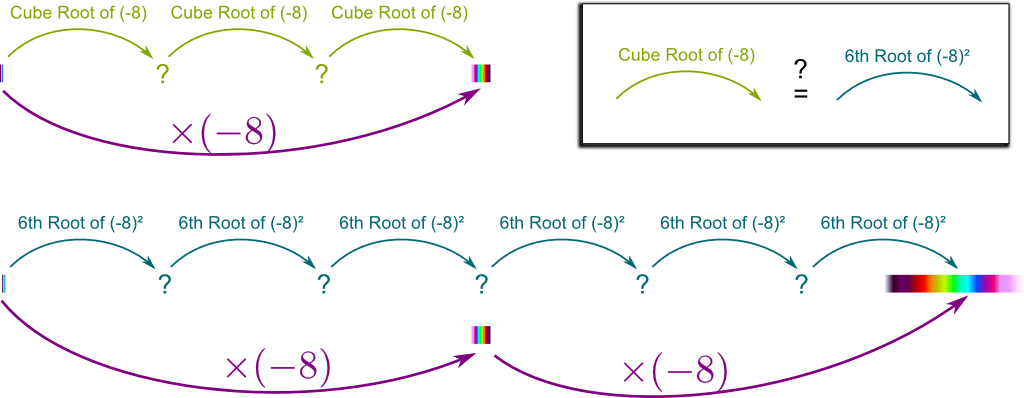As you can deduce it from the figure above, any cube root of $(-8)$ is also a 6th root of $64$. Indeed, applying the green operation six times will necessary be equivalent to applying “$\times (-8)$” twice. But some of the 6th roots of $64$ aren’t cube roots of $(-8)$! It’s not too hard to prove that these are the cube roots of $8$, the other square root of $64$. I invite to do that as an exercise!

Now, by denoting $\sqrt{-8}$ the set of all cube roots of $-8$ and $\sqrt{64}$ the set of all 6th roots of $64$, we can elegantly correct the paradox! These notations are highly non-conventional and I have been blamed for using them. But I believe it to provide an insightful and beautiful solution to the paradox. Also, if you can make the difference between $n$-th roots and the classical notation $\sqrt[n]{x}$ for $x \geq 0$, then you’ll have made a huge breakthrough in the understanding of $n$-th roots.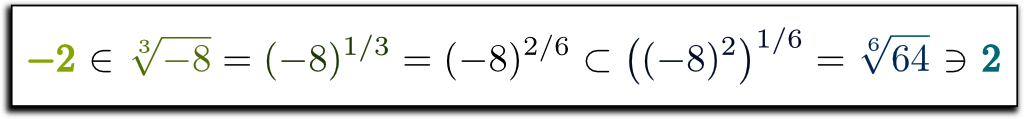Granted, this could have been proved without involving geometrical operations, as the key aspect is the property of groups in pure algebra, which the geometrical operations form. But I think it’s much more insightful when you consider this equation for geometrical operations.
Using our notations of $n$-th roots as defining the set of all $n$-th roots, note that, if $n/m$ is not an irreducible, we have $(a^{n})^{1/m} \neq (a^{1/m})^n$. For instance, when $a=1$, $n=m=2$, we have $(1^2)^{1/2} = \{1, -1\}$, while $(1^{1/2})^2 = \{(-1)^2, 1^2\} = \{1\}$. One natural way to define $a^{n/m}$ would then be $a^{n/m} = (a^{1/m})^n$. I’ll leave you as an exercise to prove that $a^q$ would then be well-defined for any $q \in \mathbb Q$.

## Homotheties and Rotations

Although insightful, the descriptions of complex numbers we have given so far aren’t very rigorous.

So what’s the rigorous definition of complex numbers?

From a geometrical perspective, complex numbers should actually regarded as a certain collection of transformations of a plane rather than those of a line. This plane is called the complex plane. It is infinite in all directions and has a unique center, called the origin. Plus, one of its axis which goes through the origin is known as the real number line. The axis perpendicular to the real number line is known as the imaginary number line.

So what kind of transformations of the plane are we talking about?

The transformations which corresponds to a complex numbers are those we have been using so far: homotheties and rotations centered on the origin. These two operations are the symmetries described below: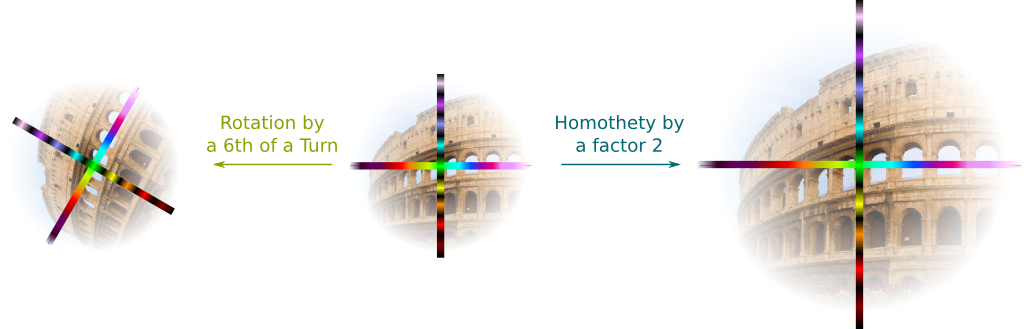The key aspect of these operations is that any number of rotations and homotheties can be combined, and that the order in which they are combined does not matter. In technical terms we say that all these geometrical transformations are associative and commutative. Another important fact is that all usual numbers can be matched uniquely with one such geometrical operation. For instance, what’s the operation corresponding to the number $2$?

And I guess that it corresponds a homothety by a factor 2…

Yes, which is also known as “$\times 2$”! What about the number $(-1)$?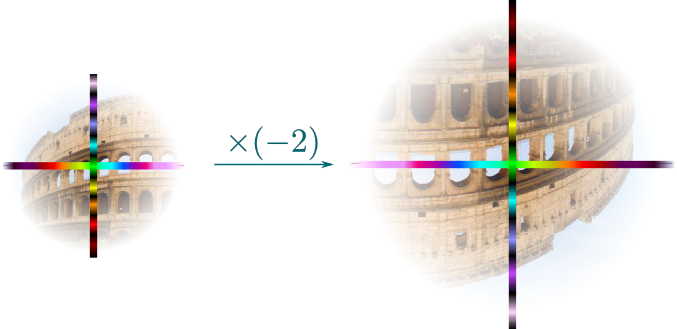The operation “$\times (-1)$” inverted the number line… So I guess it’s a symmetry along the imaginary axis!

Nope… Keep in mind that we can only use homotheties and rotations!

Arg… Humm… I know! It’s a half-turn rotation!

Excellent! Let me give you one last example: $(-2)$ is a homothety of a factor 2 combined with a rotation of a half turn. Now, more generally, any combination of a homothety and a rotation forms a complex numbers.

Wait… A complex number?

Yes! Now, the homothety is defined by a positive factor called module and is commonly denoted $\rho$. By convention, the rotation is defined by an angle of anti-clockwise turn called argument and is often denoted $\theta$. Since these two parameters uniquely define the combination of a homothety and a rotation, each complex number can be represented by the couple $(\rho, \theta)$.

In terms of pure algebra, we are here defining the set of complex numbers by $\mathbb C = (\mathbb R_+^* \times SO(2)) \cup \{0\} = (\mathbb R_+^* \times \mathbb R/\tau \mathbb Z) \cup \{0\}$. As a topological group, $\mathbb C^* = \mathbb C – \{0\}$ is then trivially isomorphic to $\mathbb R_+^* \times \mathbb S^1$, where $\mathbb S^1 = \mathbb R/\mathbb Z$ is the circle. A clever extension of this approach can then be defined to construct the set of quaternions $\mathbb H$, which nearly equals $(\mathbb R_+^* \times SO(3)) \cup \{0\}$ (technically, the “rotations” of $\mathbb H$ form a double covering of $SO(3)$). Quaternions are a bit more complicated though, as they are not commutative. Indeed, as you can see it if you play Rubik’s cube, two rotations in space do not commute in general. If you can, please write about quaternions!
And any number is a complex number?

Well, as we’ve said, any positive number is just a homothety. This includes a rotation of angle $0$. Thus, any positive number $x$ is the complex number $(x, 0)$. Now, if $x$ is a positive number, then $(-x)$ corresponds to a homothety of factor $x$, and of a half turn. Since a half turn corresponds to angle $\pi$, the number $(-x)$ is thus the complex number $(x, \pi)$.

What? A half turn is $\pi$? Shouldn’t we rather give the full turn a name like $\tau$, and call the half turn $\tau/2$?

I know! Some mathematicians even think that $\pi$ should be withdrawn from all equations to be replaced by $\pi = \tau/2$. There’s even a manifest supporting that… as you can see it in the following awesome video by ViHart:

I personally much prefer $\tau$ over $\pi$… Since you’ve probably learned $\pi$, I’ll try to insert it in this article, but I’ll be doing most of it with $\tau$. In particular, note that if $x > 0$, then $(-x)$ is the complex number $(x, \tau/2)$.

Humm… Good remark. We need a new transformation which corresponds to zero! This transformation consists in collapsing the whole complex plane onto its origin.

One thing troubles me… You’ve been saying that complex numbers are geometrical transformations? In what possible sense are they numbers?

Not in an obvious one for sure! But, for one thing, we can multiply complex numbers. This corresponds to performing successively the geometric operations associated to the complex numbers. And what’s beautiful is that this has an algebraic translation! What I mean by that is that multiplying $(\rho_1, \theta_1)$ by $(\rho_2, \theta_2)$ corresponds to two homotheties by factors $\rho_1$ and $\rho_2$ and two rotations of angles $\theta_1$ and $\theta_2$. Now, two homotheties of factors $\rho_1$ and $\rho_2$ combine into a homothety of factor $\rho_1 \times \rho_2$, while two rotations of angles $\theta_1$ and $\theta_2$ result in a rotation of angle $\theta_1+\theta_2$. Thus, we have the product $(\rho_1, \theta_1) \times (\rho_2, \theta_2) = (\rho_1 \times \rho_2, \theta_1 + \theta_2)$. How sweet is that?

Note that angles are defined up to $\tau$, which means that the angle $\theta+\tau$ is the same as $\theta$. But let’s not dwell too much on modular algebra. If you can though, please write about it! Now, if you are familiar with modular algebra, then note that, in pure algebra terms, what we’ve done here is defining the group $(\mathbb C^*, \times)$ as the product group $(\mathbb R_+^*, \times) \times (\mathbb R /\tau \mathbb Z, +)$.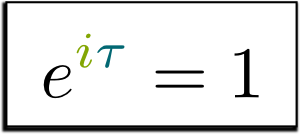For reasons I won’t be dwelling on here, Leonhard Euler showed that it made sense to write the complex number $(\rho, \theta)$ as $\rho e^{i\theta}$. Given this writing, the multiplication of two complex numbers follows the usual laws of algebra, as $(\rho_1 e^{i\theta_1}) \times (\rho_2 e^{i\theta_2}) = (\rho_1 \rho_2) e^{i(\theta_1+\theta_2)}$. Also, plugging in $\rho=1$ and $\theta=\pi$, we obtain the equality $e^{i\pi} = (1, \pi) = -1$, which is usually rewritten as $e^{i\pi} + 1=0$. This beautiful formula is known as Euler’s identity! But, in spite of probably angering the Swiss scholar, I’d rather have it written with $\tau$ as $e^{i\tau} = 1$. This last formula is much more insightful, as it says that $\tau$ represents a full turn.

To understand why complex numbers can be written $\rho e^{i\theta}$, check my article on Euler’s identity!
I guess this is a nice construction, but I don’t see the point…

The ability complex numbers have to describe rotations is the reason why they are so much used in oscillating problems in physics and engineering. Instead of involving ugly trigonometry, the function $f(t)=e^{it}$ provides an elegant description of these motions, which greatly facilitates computations! But that’s still just the tip of the iceberg. To unveil the true magic of complex numbers, we’ll need to dig deeper!

## Points in the Complex Plane

In the 19th century, German mathematician Carl Friedrich Gauss, the Prince of mathematics, provided a powerful visualization of complex numbers. To get there, notice the incredible fact that $1 \times x=x$ when $x$ is a number. Thus, if I show you a geometrical transformation “$\times x$”, then you can easily find which $x$ I chose by looking at what point the number $1$ is sent to. Similarly, if I give you a geometrical transformation $(\rho, \theta)$, then you can find out the values of $\rho$ and $\theta$, as they’ll be the polar coordinates of the point $1$ is sent to! This point is called the image of 1.

The polar coordinates? Can you give an example?

Sure! Below is the the combination of a homothety by a factor 2 and a rotation by an angle $2\tau/3$ (2 thirds of a turn).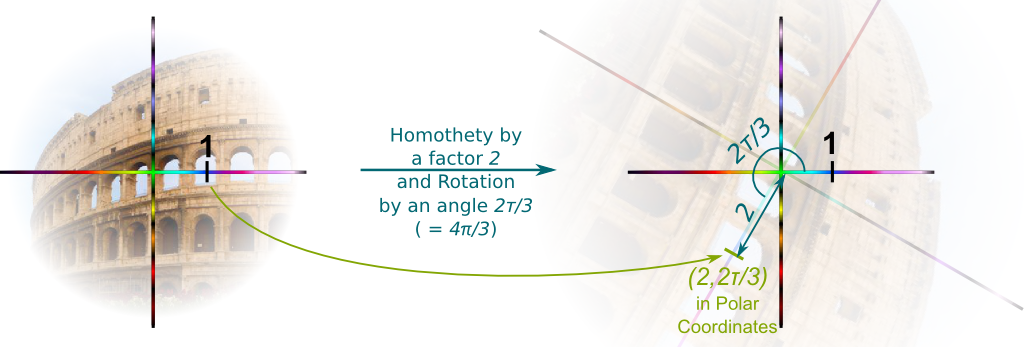The factor of homothety $\rho$ is the distance between the image of 1 and the origin, while the angle of rotation $\theta$ is the (anti-clockwise) angle from $1$ to its image.

These look like the polar coordinates!

Exactly! This shows that any geometrical transformation can be translated into a point in the complex plane whose polar coordinates are given by the factor of homothety and the angle of rotation! And this is a one-to-one correspondence between geometrical transformations and points! Thus, we can identify geometrical transformation with points in the complex planes.

In pure algebra terms, what we’ve unveiled here is a natural bijection between $\mathbb R_+^* \times SO(2)$ and $\mathbb R^2-\{0\}$. This bijection is a homeomorphism! Plus, it is naturally extended to a homeomorphism $(\mathbb R_+^* \times SO(2)) \cup \{0\} \rightarrow \mathbb R^2$. Thus, we can identify both sets, and we call them both $\mathbb C$.
Could we translate these coordinates back in classical Cartesian ones?

Yes! But before doing that, let’s first look to which point of the complex plane the geometrical transformation $i$ is associated to: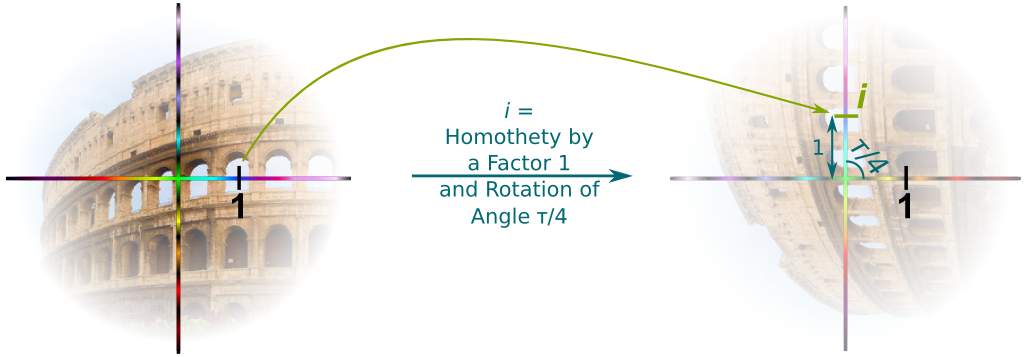So, $i$ is the point right above the origin? That’s funny…

I know! What’s also particularly interesting is that we can now describe Euclidean planar geometry with complex numbers!

## Vectors in the Complex Plane

To complete the construction of complex numbers, we need to associate any point in the complex plane with a vector.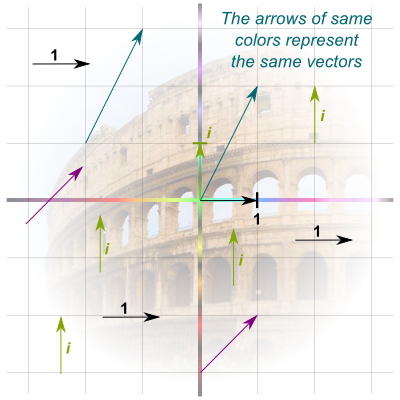A vector? What the hell is that?

A vector is a motion in the complex plane. This motion is often represented by an arrow from an initial point to a final point. But what’s important to keep in mind is that the vector corresponds to the motion, not the arrow. Two arrows may correspond to the same motion even though they don’t start at the same initial points, as displayed by the arrows of same colors in the figure on the right.

So how do we associate any point in the complex plane with a vector?

Given a point in the complex plane, we can draw the arrow from the origin to that point. This vector associated to this arrow will then be the vector associated to the point in the complex plane. For instance, $i$ is associated to the arrow from $0$ to $i$, which corresponds to the green arrows in the figure on the right.

I think I get it… But what’s the point in mapping points to vectors?

We can now define the addition of complex numbers!

How do we do that?

By combining the motions associated to the vectors! For instance, combining the purple and green motions is a motion of one unit to the right and by two units upwards. This is equivalent to the blue motion only! This means that $purple + green = blue$. And this can be visualized geometrically by having the purple and blue arrows starting at the same point, while the green arrow is put at the end of the purple arrow. The purple, green and blue arrows must then form a triangle, as done below: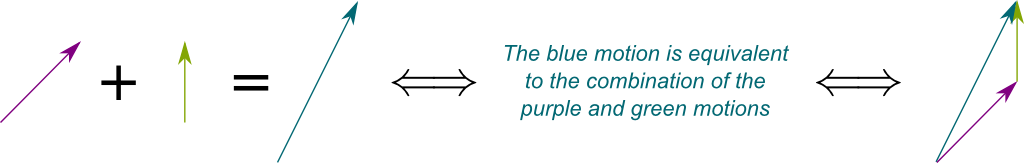And since all vectors correspond to a complex number, we can now do additions of complex numbers by adding their corresponding vectors!

Cool!

Now here comes the key part. All vectors can be decomposed uniquely as a sum of $1$ and $i$. For instance, the purple vector can be obtained by a combination of the vector associated to $1$, and a vector associated to $i$. Thus, $purple = 1+i$. Similarly, the blue vector is a combination of $1$ and two times $i$. Hence, $blue = 1+2i$.

So all vectors are a certain number of times $1$ plus a certain number of times $i$?

Exactly! And since all vectors correspond to a complex number, all complex numbers can thus be written $a+bi$, where $a$ and $b$ are usual numbers. This decomposition enables simple computations of additions of complex numbers! Indeed, if you consider any two complex numbers $z_1$ and $z_2$, then we know by now that each can be decomposed as $z_1 = a_1+b_1i$ and $z_2 = a_2 + b_2 i$. The sum of $z_1$ and $z_2$ is then given by $z_1 + z_2 = (a_1+b_1i) + (a_2+b_2i) = (a_1+a_2) + (b_1+b_2)i$.

I should also mention that each complex number $z$ is also associated with an operation “$+z$” on the points of the complex plane. Geometrically, this operation consists in a translation of a vector which is the one that $z$ corresponds to. In fact, this mapping of $z$ to “$+z$” is an isomorphism of Euclidean vector space between the space of complex numbers and the set of translations of the complex plane.

This Cartesian description of complex numbers would have been quite useful if it hadn’t been for the more general approach of linear algebra to define vectors.

In pure algebra terms, what we’ve done here is unveiling a natural isomorphism of Euclidean vector space between $\mathbb C$ and $\mathbb R^2$. In particular, this gives the space $(\mathbb C, +)$ a structure of commutative group. This isomorphism is trivial for the classical construction of complex numbers, but it’s quite impressive in the construction of this article! Recall that we introduced $\mathbb C$ as combinations of homotheties and rotations!

## The Field of Complex Numbers

Let’s sum up what we’ve discussed so far. The awesomeness of complex numbers is that they can be identified with several different mathematical objects. They can be seen as combinations of homotheties and rotations of the complex plane, as points in the complex plane and as vectors in the complex planes. The first understanding of complex numbers describes the multiplication, while the third describes the addition. Thinking about each of the meanings of complex numbers separately is already quite mesmerizing, but the truly mind-blowing property of complex numbers occurs when we mix them!

What do you mean?

Let’s see what happens when we both have a multiplication and an addition! In particular, let’s focus on the simplest possible case, namely $(x+y) \times z$, where $x$, $y$ and $z$ are all complex numbers.

Humm… I don’t know where to start!

Well, the expression starts with the addition of $x$ and $y$…

OK… So to do the addition, we need to think of these complex numbers as vectors, right?

Exactly! Let’s draw $x$, $y$, and their sum $x+y$. But then, we need to multiply these terms by $z$. How do we do that?

I know! We need to think of $z$ as $\times z$, which is a combination of a rotation and a homothety!

Very good! This means that $xz$, $yz$ and $(x+y)z$ will be the image by the geometrical transformation “$\times z$” of $x$, $y$ and $z$. This is what’s drawn below: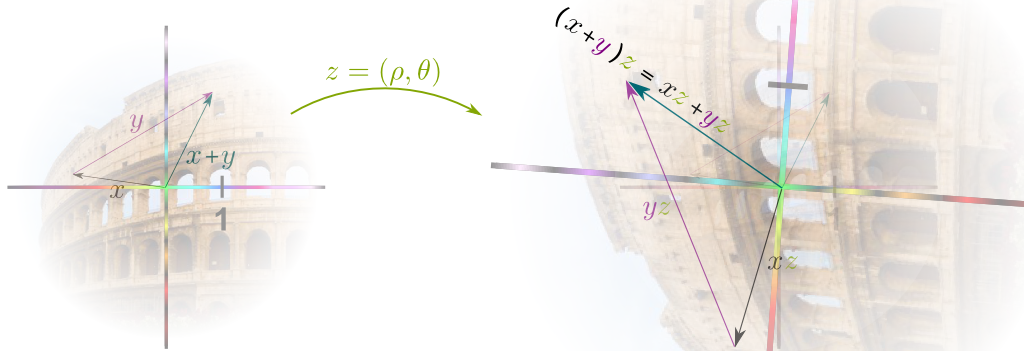Now, the magic occurs when we notice that any geometrical transformation $\times z$ preserves the shapes of triangles. As a result, the triangle $x$, $y$, $x+y$ gets transformed into the triangle $xz$, $yz$, $(x+y)z$. And this means that the sum of the sides $xz$ and $yz$ equals the last side $(x+y)z$! In other terms, $xz+yz=(x+y)z$. This is the essential distributivity property which binds the two operations we have defined on complex numbers! It says that the structure of complex numbers is much richer than the structures of geometrical operations and vectors alone.

I’m not sure I see what’s so great about that…

What’s awesome about that is that all the algebraic manipulations you could do with usual numbers still hold for complex numbers. In particular, operations like $(x+y)^2 = x^2+2xy+y^2$ are still valid for complex numbers! This strong resemblance of manipulations is what led mathematicians to call complex numbers… numbers. In pure algebra terms, we say that complex numbers form a field.

## Conclusion

To recapitulate, a complex number is very complicated mathematical object, which can be seen through diverse angles. Mainly, it can be seen as a combination of a homothety and a rotation, as a point in the complex plane or as a vector of dimension 2. These three interpretations are displayed below.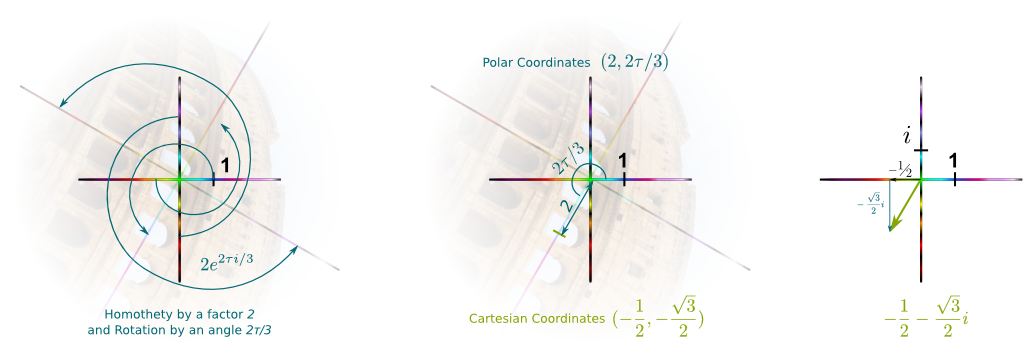But what makes complex numbers so special isn’t the different angles through which they can be seen, but the combination of them all, in sort of the same way that quantum objects aren’t simply classical waves nor classical particles. Namely, the full nature of complex numbers is unveiled as they are considered as a field. In particular, it is to that field that the fundamental theorem of algebra gets applied.

What’s that theorem?

This theorem, which was first proven by Carl Friedrich Gauss, states that all polynomial complex equations have solutions. It’s as simple as that. This property is also known as the fact that the complex numbers form the algebraic closure of real numbers. In fact, as mentioned briefly in my article on the construction of numbers, the most insightful and natural (but also terribly abstract) way to construct complex numbers is precisely by defining them as the algebraic closure of real numbers.

In pure algebra terms, let’s denote $\mathbb R[X]$ the ring of polynomials. Then, it can be shown that $(X^2+1)\mathbb R[X]$ is a maximal ideal. The space of complex numbers is then defined as $\mathbb C = \mathbb R[X]/(X^2+1)\mathbb R[X]$, and it is thus a field. This is so beautiful that I nearly cried when I first saw that!
What’s the point of this theorem?

Many more equations can now be solved! And I’m not only talking about the polynomial equations. More importantly, natural and simple solutions appear in differential equations, electromagnetism, eigen-value search, Fourier transform and number theory among many other fields. In particular, complex numbers have turned out to be the right structure to describe particle physics! Check my article on the dynamics of the wave function in quantum mechanics to see the complex numbers in action!

## More on Science4All

The Magic of AlgebraBy Lê Nguyên Hoang | Updated:2016-02 | Views: 3830
The power of algebra lies in abstraction, and abstraction is basically forgetting. By retracing the History of algebra from its roots to more recent advancements, this article unveils the numerous breakthrough in our understanding of the world, by abusing of the power of forgetting.

Construction and Definition of NumbersBy Lê Nguyên Hoang | Updated:2016-02 | Views: 9096
Although they have been used for thousands of years, an actual definition of numbers was given less than a century ago! From the most fundamental level of set theory, this article takes you to the journey of the construction of natural, integer, rational, real and complex numbers.

Numbers and ConstructibilityBy Lê Nguyên Hoang | Updated:2016-02 | Views: 9225
Last summer, I got to discover Morellet's artwork on inclined grids. Amazingly, this artwork is a display of the irrationality of $\sqrt{2}$! It's also a strong argument for the existence of this number. In this article, after discussing that, I take readers further by discussing what numbers can be constructed geometrically, algebraically, analytically or set theoretically using the power of mathematics!

Symmetries and Group TheoryBy Lê Nguyên Hoang | Updated:2016-02 | Views: 5208
Beauty is extremely hard to define. Yet, physicists and artists seem to agree on an important feature of beauty, created by mathematicians: Symmetries. This article aims at introducing the beauty and the concepts on symmetries, from the basic geometrical symmetries to the more abstract fundamental automorphisms.

The Most Beautiful Equation of Math: Euler's IdentityBy Lê Nguyên Hoang | Updated:2016-01 | Views: 93600
In 1988, Euler's identity was elected most beautiful theorem of mathematics. It has been widely taught worldwide. But have you ever stopped to really sense the meaning of this incredible formula? This article does.

Dynamics of the Wave Function: Heisenberg, Schrödinger, CollapseBy Lê Nguyên Hoang | Updated:2016-02 | Prerequisites: The Essence of Quantum Mechanics, Imaginary and Complex Numbers, Linear Algebra and Higher Dimensions | Views: 10660
On one hand, the dynamics of the wave function can follow Schrödinger equation and satisfy simple properties like Heisenberg uncertainty principle. But on the other hand, it can be probabilistic. This doesn't mean that it's totally unpredictable, since the unpredictability is amazingly predictable. Find out how these two dynamics work!

## One comment to “Imaginary and Complex Numbers”

1.henning says:

Inspired introduction to complex numbers! Introducing it that way will nicely pave the way for geometric algebra.

Will certainly use this when teaching my kids this concept – only quibble when you write that an “anti-clockwise quarter-of-a-turn rotation [is represented] as i” you illustrate this with the horizontal and then rotated vertical number lines. So far so good, but because the transition arrow is curved, I at first thought it indicated the wrong orientation of the rotation, took me a moment to realize that this is of course not the assigned meaning. May be better to use a straight arrow for that graphic.